# Need to write excel formula

How do i write in a cell below excel formula
From G2 cell i need write.
“=IF(C2>20000000,“Hight”,“Low”)”

You can try with this syntax

``````=IF(B9>15,"HIGH","Low")
``````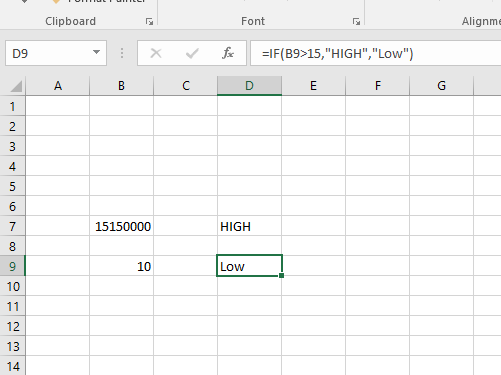Try with this syntax in the Write cell activity

``````"=IF(B9>15,'HIGH','Low')"
``````

Regards
Gokul

1 Like

Try with this one @Pujari_Manjunatha

Hello @Pujari_Manjunatha
Try this

``````"=IF(B8>15,""HIGH"",""Low"")"
``````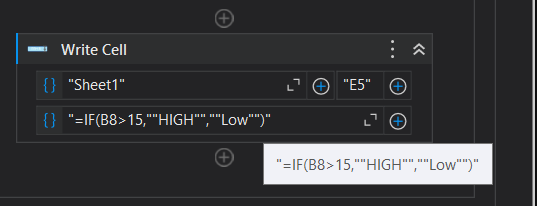Formula working fine, Then i have write for all the rows, how to do?

You have to generate the Cell reference(“B1”, “B2”, etc) and formula reference dynamically using foreach loop for the entire table

For each or for each row?

Please feel free to try both. But in this case for each row is a better fit

Is it possible for you to use Modern Excel Activities, If so, You could check the below implementation :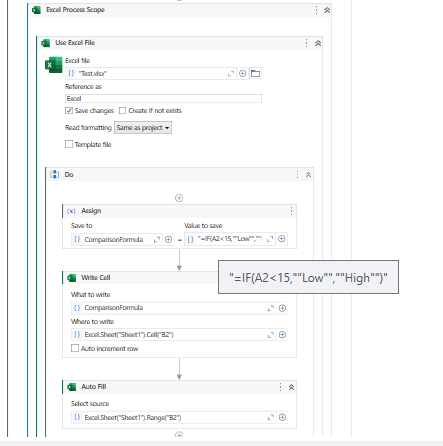We first use `Write Cell` Activity with the Formula you would want to write for the First Row Cell (B2). Then we perform Auto Fill range using the `Auto Fill` Activity. This will apply the formula to all the rows available.

Before :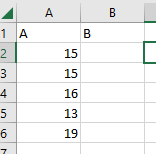After :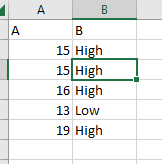Let us know if this doesn’t work or if you are facing any difficulties.

Hello @Pujari_Manjunatha
Use Excel auto fill range activity,

1. Use write cell and enter the formula, like in G2
``````"=IF(B8>15,""HIGH"",""Low"")"
``````
1. Use auto fill range activity, where pass the Source as G2 and destination as G2:G10 or your specific destination. The sheet name can change in Properties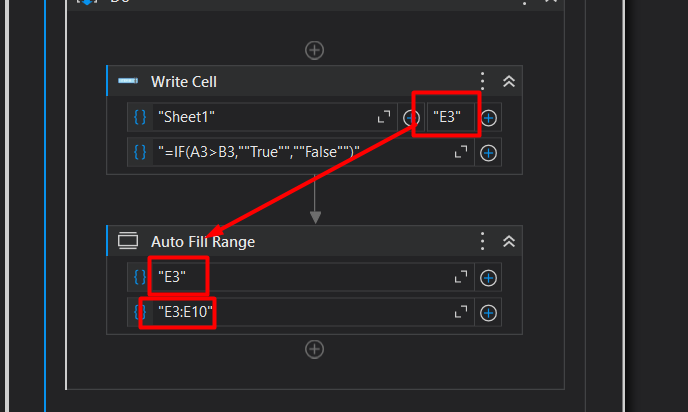Even though I can enter the formula in each cell, it always outputs the same result.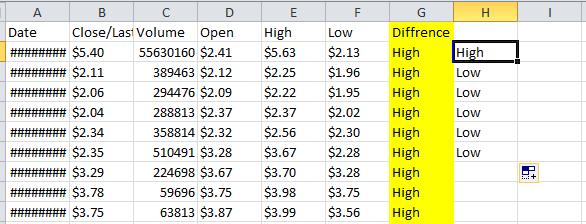@Pujari_Manjunatha
It Happens because, all rows compare with C2. We need to increment the the C range also

Try this

``````"=IF(C"+(index+2).tostring+",""High"",""Low"")"
``````
1 Like

This topic was automatically closed 3 days after the last reply. New replies are no longer allowed.